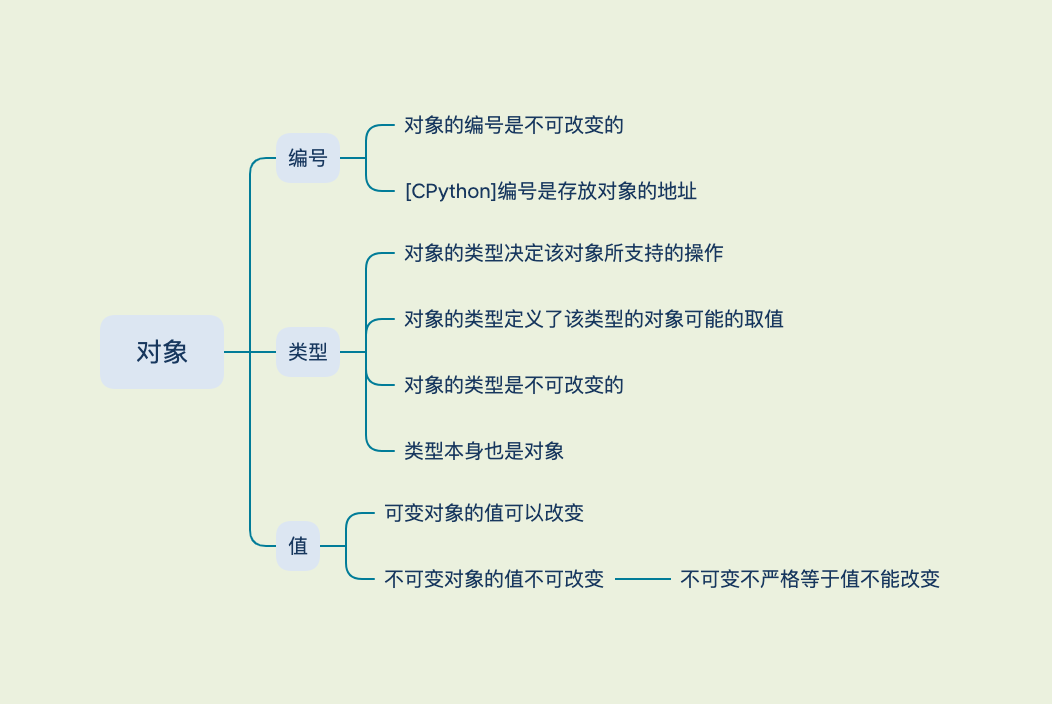# Python 面向对象

## Python 中的对象

• Python 中一切皆对象
• 对象 是对数据的抽象
• 对象 拥有编号(id)、类型(type)、值## Python 面向对象的特性

### 封装

#### 访问限制

• Python 对属性权限的控制是通过属性名来实现的，如果一个属性由双下划线开头 `__`，该属性就无法被外部访问。

• 但是，如果一个属性以 `__xxx__` 的形式定义，那它又可以被外部访问了，以 `__xxx__` 定义的属性在 Python 的类中被称为特殊属性，并且通常我们不应该把普通属性用 `__xxx__` 定义。

• 以单下划线 `_` 开头的属性 `_xxx` 虽然也可以被外部访问，但是按照约定，他们不应该被外部访问。另外该属性不能被子类继承，也不会被 `from module import *` 导入

关于 Python 中下划线用法的总结可参考
https://dbader.org/blog/meaning-of-underscores-in-python

### 继承

#### 定义新的 Student 类继承已定义的 Person 类

• 一定要用 `super(Student, self).__init__(name, gender)` 去初始化父类，否则，继承自 `Person``Student` 将没有 `name``gender`
• 函数 `super(Student, self)` 将返回当前类继承的父类，即 `Person`，然后调用 `__init__()` 方法，注意 `self` 参数已在 `super()`中 传入，在 `__init__()` 中将隐式传递，不需要写出（也不能写）
• 初始化父类的属性后，只需要再把子类额外的属性加上
``````# Person 类
class Person:
def __init__(self, name, gender):
self.name = name
self.gender = gender

# 继承 Person 的 Student 类
class Student(Person):
def __init__(self, name, gender, score):
super(Student, self).__init__(name, gender)
self.score = score
``````

#### 多重继承

• D 同时继承自 B 和 C，也就是 D 拥有了 A、B、C 的全部功能。多重继承通过 `super()` 调用 `__init__()` 方法时，A 虽然被继承了两次，但 `__init__()` 只调用一次。
• 多重继承的目的是从两种继承树中分别选择并继承出子类，以便组合功能使用。
``````graph BT
subgraph 菱形继承
B --> |继承| A
C --> |继承| A
D --> |继承| B
D --> |继承| C
end
``````
``````class A:
def __init__(self, a):
print("init A...")
self.a = a
print("end A...")

class B(A):
def __init__(self, a):
print("init B...")
super(B, self).__init__(a)
print("end B...")

class C(A):
def __init__(self, a):
print("init C...")
super(C, self).__init__(a)
print("end C...")

class D(B, C):
def __init__(self, a):
print("init D...")
super(D, self).__init__(a)
print("end D...")

d = D(1)

# 调用结果
init D...
init B...
init C...
init A...
end A...
end C...
end B...
end D...
``````

### MRO 问题

``````class A:
pass

class C(A):
pass

class B(A, C):
pass

B()

# 调用结果
...
TypeError: Cannot create a consistent method resolution
order (MRO) for bases A, C
``````

### 多态

• 继承
• 重写

Python 作为一种动态语言，崇尚鸭子类型鸭子类型，参数在传入之前无法确定也不需要声明参数类型，可以像多态一样工作但无需继承，并支持实现多态性。